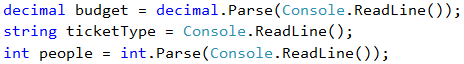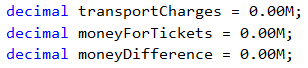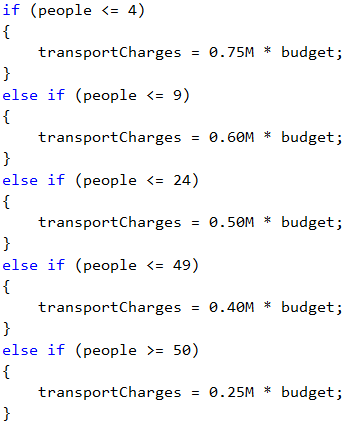Problem: Game Tickets

A group of football fans decided to buy tickets for Euro Cup 2016. The tickets are sold in Bulgarian leva (BGN) in two price categories:

• VIP499.99 BGN (Bulgarian leva)
• Normal249.99 BGN (Bulgarian leva)

The football fans have a shared budget, and the number of people in the group determines what percentage of the budget will be spent on transportation:

• 1 to 4 – 75% of the budget
• 5 to 9 – 60% of the budget
• 10 to 24 – 50% of the budget
• 25 to 49 – 40% of the budget
• 50 or more – 25% of the budget

Write a program that calculates whether the money left in the budget will be enough for the football fans to buy tickets in the selected category, as well as how much money they will have left or be insufficient.

Input Data

The input data is read from the console and contains exactly 3 lines:

• The first line contains the budget – real number within the range [1 000.00 … 1 000 000.00].
• The second line contains the category – "VIP" or "Normal".
• The third line contains the number of people in the group – an integer within the range [1 … 200].

Output Data

Print the following on the console as one line:

• If the budget is sufficient:
• "Yes! You have {N} leva left." – where N is the amount of remaining money for the group.
• If the budget is NOT sufficient:
• "Not enough money! You need {М} leva." – where М is the amount that is insufficient.

The amounts must be formatted up to the second digit after the decimal point.

Sample Input and Output

Input Output Explanations
1000
Normal
1
Yes! You have 0.01 leva left. 1 person: 75% of the budget are spent on transportation.
Remaining amount: 1000 – 750 = 250.
Category Normal: the ticket price is 249.99 * 1 = 249.99
249.99 < 250: the person will have 250 - 249.99 = 0.01 money left
Input Output Explanations
30000
VIP
49
Not enough money! You need 6499.51 leva. 49 persons: 40% of the budget are spent on transportation.
Remaining amount: 30000 - 12000 = 18000.
Category VIP: the ticket costs 499.99 * 49.
24499.51 < 18000: the amount is not enough, more money needed: 24499.51 - 18000 = 6499.51

Hints and Guidelines

We will read the input data and perform the calculations described in the task requirements, in order to check if the money will be sufficient.

Processing the Input Data

Let's read carefully the requirements and examine what we expect to take as input data, what is expected to return as a result, as well as what are the main steps for solving the problem.

For a start, let's process and save the input data in appropriate variables:Calculating Transportation Costs

Let's create and initialize the variables needed for doing the calculations:Let's review the requirements once again. We need to perform two different block calculations.

By the first set of calculations we must understand what part of the budget has to be spent on transportation. You will notice that the logic for doing these calculations only depends on the number of people in the group. Therefore, we will do a logical breakdown according to the number of football fans.

We will use conditional statement – a sequence of if-else blocks.Calculating Ticket Costs

By the second set of calculations we need to find out what amount will be needed for purchasing tickets for the group. According to the requirements, this only depends on the type of tickets that we need to buy.

Let's use a switch-case conditional statement.Calculating Total Costs

Once we have calculated the transportation costs and ticket costs, what remains is to calculate the end result and understand if the group of football fans will attend Euro Cup 2016 or not, provided the available parameters.

For the output, in order to spare one else condition in the construction, we will assume that the group can, by default, attend Euro Cup 2016.Printing the Result

Finally, we need to display the calculated result on the console.